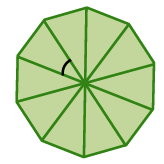### Home > INT2 > Chapter 8 > Lesson 8.2.2 > Problem8-50

8-50.

What is the area of a regular decagon if the length of each side is $20$ units?

Find the measure of a central angle.

Use this to determine the height of one of the triangles.

Find the area of one of the triangles.

Find the total area.

$3077.7$ un$^2$